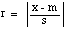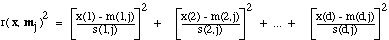Scaling

In general, the numerical value for a feature x depends on the units used, .i.e., on the scale. If x is multiplied by a scale factor a, then both the mean and the standard deviation are multiplied by a. (The variance is multiplied by a2.)

Sometimes it is desirable to scale the data so that the resulting standard deviation is unity. This is easily done: just divide x by the standard deviation s. Similarly, in measuring the distance from x to m, it often makes sense to measure it relative to the standard deviation. The so-called standardized distance from x to m is given by.

Note that r is invariant to translation and invariant to scale. This suggests an important generalization of a minimum-Euclidean-distance classifier. Let x(i) be the value for Feature i, let m(i,j) be the mean value of Feature i for Class j, and let s(i,j) be the standard deviation of Feature i for Class j. In measuring the distance between the feature vector x and the mean vector mj for Class j, suppose that we use the standardized distance.

This distance has the important property that it is scale invariant . That is, if we measure distance in this way, the units we use for the various features will have no effect on the resulting distances, and thus no effect on the final classification.